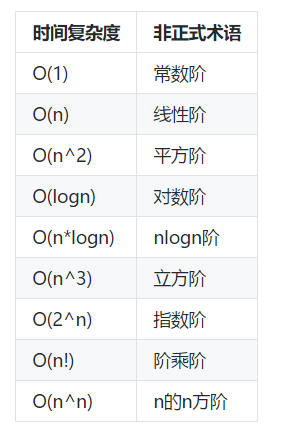# 第2章 算法天平:复杂度分析

• 算法分析方法论
• 算法的时间复杂度
• 常见的时间复杂度类型
• 时间复杂度知识扩展
• 算法的空间复杂度
• 小结

## 2.1 算法分析方法论

### 2.1.1 事后统计方案

• 首先，事后统计的时间点是算法程序完成之后。这就带来了很大的隐患。如果能达到要求还好，万一达不倒需求的效果，那就意味着之前的人力精力财力都浪费掉了，这对项目运营者可能是致命打击！再就是如果是上线运营阶段，那造成的损失就更大。
• 第二，计算机的硬件环境也严重影响着算法的性能。在不同的硬件环境上，算法的表现不一，这会掩盖掉算法本身的优势和劣势，使我们并不能客观的说是算法太好或者太坏。
• 第三，测试数据的选择本身也影响了算法的执行效果！数据的规模，数据的流量，数据的分布都会影响算法的执行，使我们也并不能客观的描述一个算法的效率和优劣。

• 算法本身的策略和方法。
• 编译产生的代码质量。
• 问题的输入规模。
• 机器指令的执行速度。

## 2.2 算法的时间复杂度

• 不考虑任何的软硬件环境！
• 假设程序每一条语句的执行时间相同，均为t;
• 只考虑最坏情况，即数据规模足够大，设为n。

### 2.2.1 时间复杂度和大O表示法

``````T(n) = O(f(n))
``````

``````f(n) = 算法执行语句的数量 * t
``````

``````T(n) = O(算法运行总时间)
``````

### 2.2.2 时间复杂度计算实例

``````计算 1+2+3+4+5.....+n 的和！
``````

#### 第一种思想：我们可以生成一个n个长度的数组，然后把数据存储到数组中，最后计算数组各项的和。

``````function sum( \$n ){
// 设定返回结果
\$res = 0;                                   //{1}
// 生成数组[1,2,3,4,5...n]
\$arr = range(1,\$n);                         //{2}

// 计算数组中各项的和
for(\$i = 0; \$i < \$n; ++ \$i){                //{3}
\$res += \$arr[\$i];                       //{4}
}

// 返回结果
return \$res;                                //{5}
}
``````

``````分析代码可知，其中{1}{2}{5}所标注的代码语句整个过程每条都只执行1次，而{3}{4}标注的语句执行过程中每一条都要执行n次！所以总条数N:

N = 2*n + 3
``````

``````前面我们已经制定了前提，以为每一条语句执行的时间实行同的，都是t，所以:

f(n) = N * t = (2*n + 3) * t
``````

``````我们这里的时间复杂度指的是最坏的时间复杂度，使用大O表示法表示:

T(n) = O( f(n) ) = O( (2*n + 3) * t )

即

T(n) = O( (2*n + 3) * t )
``````

``````实际上到这里我们已经成功的计算出了这个算法的时间复杂度，但是在实际的时间复杂度的表示中，对于上面的结果我们要做一定的简化，简化结果是:

T(n) = O(n)
``````

### Tips:简化步骤

• 1, 去掉常数t:

``````T(n) = O( (2*n + 3) )
``````
• 2, 用常数1取代运行时间中的所有加法常数:
``````T(n) = O( 2*n + 1 )
``````
• 3, 在修改后的运行次数函数中，只保留最高阶项:
``````T(n) = O( 2*n + 1 )
``````
• 4, 如果最高阶项存在且不是1，则去除与这个项相乘的常数:
``````T(n) = O( n + 1 )
``````
• 5, 如果存在数据规模n, 去掉加法常数项:
``````T(n) = O(n)
``````

## Tips

#### 第二种思想：直接循环计算数组各项的和。

``````function sum( \$n ){
// 设定返回结果
\$res = 0;                                   //{1}

// 计算数组中各项的和
for(\$i = 1; \$i < \$n; ++ \$i){                //{2}
\$res += \$i;                             //{3}
}

// 返回结果
return \$res;                                //{4}
}
``````

``````N = 2*n + 2
``````

``````f(n) = N * t = (2*n + 2) * t
``````

``````T(n) = O( f(n) ) = O( (2*n + 2) * t )
``````

``````T(n) = O(n)
``````

#### 第三种思想：利用高斯公式，f(n) = (1+n)*n/2。

``````function sum( \$n ){
// 设定返回结果
\$res = 0;                                   //{1}

\$res = ( 1 + \$n ) * \$n / 2;                 //{2}

// 返回结果
return \$res;                                //{3}
}
``````

``````N = 3
``````

``````f(n) = N * t = 3 * t
``````

``````T(n) = O( f(n) ) = O( 3 * t )
``````

``````T(n) = O(1)
``````

``````假如计算机一秒钟可以运行一条语句，如果计算的是从1到100的和，那么前两种时间复杂度是O(n)级别的算法应该在100秒完成计算(当然现实中要快的多), 而第三种算法需要1秒钟！如果计算的数量是从1到1000000的和，第一种和第二种O(n)级别的算法要运行1000000秒，而第三种需要1秒！
``````

• 1，同一问题的不同算法可以通过时间复杂度来比较效率优劣！
• 2，对于一个算法可以通过时间复杂度来了解随着数据规模渐变而影响算法的效率变化情况！

ok, 下面就让我们来看看有哪些常见的时间复杂度级别，并且让我们类对比这些级别的时间复杂度来更深入的学习时间复杂度的本质！

## 2.3 常见的时间复杂度`````` O(1) < O(logn) < O(n) < O(nlogn) < O(n^2) < O(n^3) < O(2^n) < O(n!) < O(n^n)
``````

## 2.4 时间复杂度知识扩展

### 2.4.1 最好情况下的算法时间复杂度

``````最好情况下的时间复杂度又叫做 最好时间复杂度，使用大Ω表示法表示！
``````

### 2.4.2 平均情况下的算法时间复杂度

``````平均情况下的时间复杂度又叫作 平均时间复杂度, 使用大Θ表示法表示！
``````

“平均时间复杂度”也有一定的实用性，它描述了一个算法在处理数据过程中单个数据元素花费的平均时间！但是“平均时间复杂度”有一个问题就是并不好测量，因为平均时间是在真正运行过程中统计并且计算出来的！但也更贴合实际效果！所以这种表示方法很难通过直接评估得到结果！这个更加接近事后统计的一种方案。

``````( 1+2+3+4+...100 ) / 100 = 50.5
``````

## 2.5 算法的空间复杂度

### 2.5.1 空间复杂度的表示

``````算法的空间复杂度实际上描述的就是算法程序运行时占用的内存大小！记作:

S(n)=O( f(n) )

``````

``````//代码片段1

\$arr = [1,2,3,4,5];

for( \$i=0; \$i < count( \$arr ); \$i ++ ){
echo \$arr[ \$i ];
}
``````

``````//代码片段2

\$arr = [1,2,3,4,5];
\$len = count( \$arr );

for( \$i=0; \$i < \$len; \$i ++ ){
echo \$arr[ \$i ];
}
``````

### 2.5.2 空间复杂度的计算

#### 代码片段一的空间复杂度的计算

``````function arrIterator( \$arr ){                   {1}

for( \$i=0; \$i < count( \$arr ); \$i ++ ){     {2}
echo \$arr[ \$i ];                        {3}
}

}
``````

``````分析代码可知，其中用到的变量如下：
\$arr    数组    存储有n个整型元素
\$i      整型
``````

``````    f(n) = n * 8 + 8 = 8*n + 8
``````

``````    S(n) = O( f(n) ) = O( 8*n + 8 )

即

S(n) = O( 8*n + 8 )
``````

``````简化方法和时间复杂度相同:

S(n) = O(n)
``````

### Tips

#### 代码片段二的空间复杂度的计算

``````function arrIterator( \$arr ){                   {1}

\$len = count( \$arr );                       {2}

for( \$i=0; \$i < \$len; \$i ++ ){              {3}
echo \$arr[ \$i ];                        {4}
}

}
``````

``````分析代码可知，其中用到的变量如下：
\$arr    数组    存储有n个整型元素
\$len    整型
\$i      整型
``````

``````    f(n) = n * 8 + 8 + 8 = 8*n + 16
``````

``````    S(n) = O( f(n) ) = O( 8*n + 16 )

即

S(n) = O( 8*n + 16 )
``````

``````    S(n) = O(n)
``````